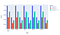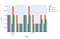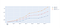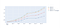# Blending Signals for Portfolio Construction

## Benefits of portfolio diversifications

Signal combinations are important in many fields, it is also important in portfolio construction, let’s find out why.

Not always

Suppose I have a portfolio (1) that returns 1% on even days and 0.5% on odd days:

Suppose I have another portfolio (2) that produces returns which alternate between 0.5% and 0.25%:

Should I diversify and invest in both portfolios?

Both portfolios are exactly the same, except the second portfolio returns less than the first portfolio, so it would seem redundant to invest in both.

To quantify this, let’s take a look at the average daily returns and the volatilities of the two portfolios and the result of investing in both (50% each):Daily Returns of Portfolio 1, 2 and combined (Image By Author)

The green bars in the figure above are the daily returns of investing equally in portfolio 1 and 2. Clearly the combined portfolio returns more than portfolio 2 but less than portfolio 1.

In fact, it makes no difference which portfolio you invest in if you had access to leverage, because the information ratio of the three portfolios are the same:

IR = average daily returns / volatility

IR of portfolio 1, 2, 1+2 = 45.18

When does it make sense to diversify?

Let’s take a look at another example.

Suppose I have a new portfolio (4) with the following daily returns from January 1st to January 14th:

And another portfolio (5) with the following daily returns:

Should I invest in one portfolio or the other? Or should I invest in both?Daily Returns of Portfolio 4, 5 and combined (Image By Author)

From looking at the plot, it seems that the combined portfolio has slightly lower returns than portfolio 5, but with less volatility. Taking a look at the sharpe ratios:

It looks like combining the two portfolios produces the best sharpe!

Taking a look at the cumulative returns of the 3 portfolios:Cumulative Returns of Portfolio 4, 5 and combined (Image By Author)

We can see that the combined portfolio, which having returns lower than portfolio 5, also has lower volatility:

If we leverage the combined portfolio to the same level of volatility as portfolio 5 ( std(return 4)/ std(returns 4+5) = 1.42), the returns of the combined portfolio would be superior:Cumulative Returns of Portfolio 4, 5, Combined, Combined with Leverage (Image by Author)

Behind the scene

Why did the blending of two portfolio work in one case (portfolio 4 and 5) but not the other (portfolio 1 and 2)?

The answer lies in the correlation of the portfolios:

For portfolio 1 and 2, the correlations between them is 1.0. Where as the correlations between portfolio 4 and 5 is close to zero (1.43e-31).

Mathematical background

The phenomenon can be understood in terms of the effect of correlation on Information Ratio:

Above are the expressions of information ratios of portfolio 1 and 2.

Information Ratio of portfolio 1+2 can be expressed as:

The information ratio of the combined portfolio, p3, is the sum of the mean returns of portfolio 1 and 2 divided by the square root of the sum of the squared standard deviations of portfolio 1 and 2 plus two times the covariance of portfolio 1 and 2.

So, take for example, two portfolios of mean returns 1 and standard deviations 2 and 3:

If their correlation is 1, then the combined portfolio has an IR of:

Smaller than the IR of portfolio 1 but bigger than the IR of portfolio 2.

If the correlation of the two portfolios is zero, then:

The IR for the combined portfolio is now 0.55, bigger than the individual portfolios.

Portfolios can benefit from diversifications, but not always, the information contained in the individual portfolios must be different (uncorrelated). Correlations between signals can also change, and look-ahead bias can cause problems as well, so careful analysis is required.

Source code:

https://github.com/swang225/data_science/blob/master/notebooks/blend_demo.ipynb

## CodeX

Everything connected with Tech & Code

Written by

## Shuo Wang

Interesting pieces on various topics in finance and technology.

Everything connected with Tech & Code

Written by

## Shuo Wang

Interesting pieces on various topics in finance and technology.

## More From Medium

Medium is an open platform where 170 million readers come to find insightful and dynamic thinking. Here, expert and undiscovered voices alike dive into the heart of any topic and bring new ideas to the surface. Learn more

Follow the writers, publications, and topics that matter to you, and you’ll see them on your homepage and in your inbox. Explore

If you have a story to tell, knowledge to share, or a perspective to offer — welcome home. It’s easy and free to post your thinking on any topic. Write on Medium